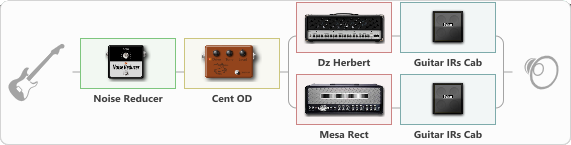# Metallica 2916

Discussion in 'ToneLib-GFX presets' started by maxim111, Feb 12, 2022.

1. Metallica 2916

Preset name: Metallica

something similar to Metallica Hardwired sound

Effects chain:Effect: "Noise Reducer" (Dynamics / Filter), active - "yes"
{
"Sens" = 87
"Mode" = Soft
}

Effect: "Cent OD" (Overdrive / Distortion), active - "yes"
{
"Drive" = 55
"Tone" = 37
"Level" = 54
}

Effect: "Splitter" (Dynamics / Filter)
{
"A-Bypass" = Off
"A-Pan" = -25
"A-Level" = 55
"B-Bypass" = Off
"B-Pan" = 25
"B-Level" = 55
"Width" = 0

'A' branch:
{

Effect: "Dz Herbert" (Amp simulators), active - "yes"
{
"Gain" = 50
"Bass" = 50
"Middle" = 70
"Treble" = 80
"Presence" = 87
"Master" = 50
"Level (dB)" = 0
}

Effect: "Guitar IRs Cab" (Cabinets), active - "yes"
{
"Model" = Mesa Rectifier (4x12")
"Mic Position" = Center
"Mic Distance" = Near
"Low Cut (Hz)" = 0
"Hi Cut (kHz)" = 20.0
"Mix" = 100
"Level (dB)" = 0
}
}
'B' branch:
{

Effect: "Mesa Rect" (Amp simulators), active - "yes"
{
"Gain" = 51
"Bass" = 44
"Middle" = 70
"Treble" = 80
"Presence" = 87
"Master" = 50
"Level (dB)" = 0
}

Effect: "Guitar IRs Cab" (Cabinets), active - "yes"
{
"Model" = Mesa Rectifier (4x12")
"Mic Position" = Center
"Mic Distance" = Near
"Low Cut (Hz)" = 0
"Hi Cut (kHz)" = 20.0
"Mix" = 100
"Level (dB)" = 0
}
}
}

Note: You will need to download and install the ToneLib-GFX software to use the preset.

#### Attached Files:

• ###### Metallica_2916.tlgfx
File size:
731 bytes
Views:
2,014
qred, renanzus228, Pat517 and 6 others like this.